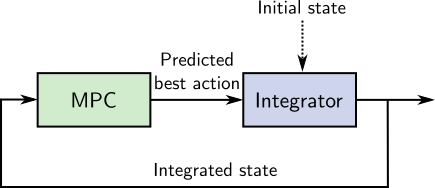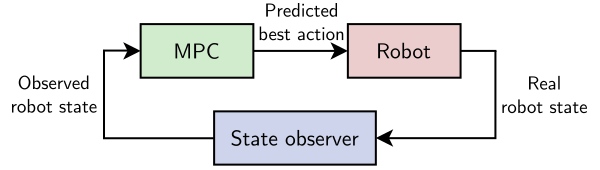# Open loop and closed loop model predictive control

There are two ways model predictive control (MPC) has been applied to legged locomotion so far: open loop and closed loop MPC. In both cases, a model predictive control (numerical optimization) problem is derived from a model of the system and solved, providing a sequence of actions that can be used to drive the actual system. Open loop and closed loop MPC differ in their choice of initial state.

## Open loop model predictive control

Open loop MPC is a motion planning solution where the plan is "unrolled" progressively. In bipedal walking, it is also known as walking pattern generation. In this approach, MPC outputs are integrated using the same forward dynamics model as the one used to derive the MPC problem itself. For instance, that model is $$\bfx_{k+1} = \bfA \bfx_k + \bfB u_k$$ in linear model predictive control. The resulting state $$\bfx_{k+1}$$ is then used as initial state for the MPC problem of the next control cycle:Note that the initial state depicted above can be either purely model-based or observed from sensors. What characterizes open loop MPC is the use of the model for integration from one step to the next. State can also be reinitialized from sensors at specific events, such as when the robot stops walking or after an impact.

One of the first breakthroughs in humanoid walking, the preview control method (Kajita et al., 2003), is open loop. It was later shown to be equivalent to linear model predictive control (Wieber, 2006). These seminal papers don't mention the integrator directly, but open loop MPC is how the generated center of mass trajectories were executed in practice on the HRP-2 and HRP-4 humanoids. This is explicit in code that was released more recently, for instance the LIPM walking controller from CNRS or the centroidal control collection from AIST.

## Closed loop model predictive control

Closed loop MPC is initialized from the latest observation:Observations, even when they are filtered, are subject to uncertainty and measurement errors, which raises new questions and edge cases to handle compared to open loop MPC. For instance, what if the MPC problem has state constraints $$\bfC \bfx_k \leq \bfe$$, but the initial state does not satisfy $$\bfC \bfx_0 \leq \bfe$$?

This question was encountered by (Bellicoso et al., 2017) in the case of ZMP constraints during quadrupedal locomotion. It is at the origin of the robust closed-loop MPC studied by (Villa and Wieber, 2017) in the case of bipedal locomotion. Closed loop MPC is also the approach followed by (Di Carlo et al., 2018) to control the base position and orientation of a walking quadruped through contact forces.

## Pros and cons

A benefit of open loop MPC, compared to its closed loop counterpart, is that it makes it easier to enforce guarantees such as recursive feasibility by choosing proper terminal constraints. Recursive feasibility is the guarantee that if the current MPC problem is feasible, then the next MPC problem (after integration) will be feasible as well. This is an important property in practice to make sure that the robot does not run "out of plan" while walking, which is dangerous if its current state is not a static equilibrium.

Open loop MPC only generates a reference state, and is therefore commonly cascaded with a walking stabilizer to implement feedback from the observed state. The main drawback of this approach is that a stabilizer is often by design more short-sighted than a model predictive controller, so that the combined system may not be general enough to discover more complex recovery strategies (re-stepping, crouching, side stepping, ...) that closed loop MPC can discover.

## To go further

Recursive feasibility is easier to enforce in open loop MPC, but it can also be achieved in closed loop. What makes it challenging at first is that the direct feedback from observations to the initial state of the MPC problem can move it too far away from the previous solution. One way to palliate this is indirect feedback, where the initial state of the subsequent MPC problem is constrained to lie around (not exactly at) the observed one. This approach may let the plan drift, with a rate parameterized by the extent of the initial state constraint, but it can also preserve recursive feasibility.

In this short overview, we mentioned measurement errors in the initial state of closed-loop MPC, but we didn't dig into the question of measurement uncertainty. This point, as well as other sources of uncertainty, can be taken into account in the more general framework of stochastic model predictive control.

On a related note:

Thanks to Nahuel Villa for his feedback on previous versions of this post!

## Discussion

You can use Markdown with $\LaTeX$ formulas in your comment.